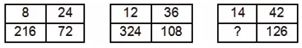# SSC Reasoning Ability Practice Questions (Day-66)

Dear Aspirants, The SSC Exams are going on at national level. Millions of candidates have eagerly prepared for the ongoing SSC exams such as CGL, CHSL, MTS, CPO, JE etc. SSC exams are usually conducted by the Staff Selection Commission to recruit the eligible candidates for various Central government departments. Mostly the selection process for all the SSC exams consists of the single or double phase of written examination based on the examination. Due to that, the written exam consisted of a certain syllabus and exam pattern. By following those regulations, the written exam has been conducted through an online mode. Based on the latest updated exam pattern and syllabus, here we have drafted the Reasoning Ability questions on a daily basis. So aspirants start your practice session with us and mould your skill in a perfect shape.

Start Quiz

1) Vikram remembers that his son Vihan’s birthday is after 25th December. Vikram’s wife remember that Vihan’s birthday is after 23rd December but before27th December. On which date of December is Vihan’s birthday?

(a) 23

(b) 24

(c) 25

(d) 26

Direction (2-3): In the following question, select the word which cannot be formed using the letters of the given word.

2) CORPORATION

(a) RATIO

(b) REPORT

(c) PORT

(d) TORN

3) PARTICULARLY

(a) RALLY

(b) TALCUM

(c) CULT

(d) PART

4) In a certain code language, “TAIL” is written as “42” and “BANT” is written as “37”. How is “GODY” written in that code language?

(a) 79

(b) 51

(c) 68

(d) 73

5) In a certain code language, “CURING” is written as “XFIRMT” and “TRACED” is written as “GIZXVW”. How is “LATELY” written in that code language?

(a) QZXHQD

(b) RXGVRC

(c) OZGVOB

(d) NXHVNB

6) If “@” denotes “multiplied by”, “\$” denotes “subtracted from”, “%” denotes “added to” and “&” denotes “divided by”, then which of the following equation mustbe true?

(a) 7 % 9 & 3 \$ 5 = 4

(b) 4 % 3 @ 6 \$ 9 = 12

(c) 3 @ 9 % 6 & 2 = 30

(d) 6 % 9 @ 2 \$ 7 = 16

7) In the following question, correct the equation by interchanging two numbers.

3 – 4 + 12 × 6 ÷ 8 = 28

(a) 3 and 4

(b) 4 and 8

(c) 3 and 8

(d) 4 and 6

8) If 42 θ 2 α 16 = 146 and 32 θ 5 α 18 = 77, then 42 θ 4 α 39 = ?

(a) 108

(b) 125

(c) 203

(d) 184

9) If 6 A 7 B 3 = 60 and 11 A 9 B 6 = 165, then 10 A 11 B 5 = ?

(a) 105

(b) 160

(c) 165

(d) 180

Direction (10): In the following question, select the number which can be placed at the sign of question mark (?) from the given alternatives.

10)(a) 178

(b) 252

(c) 378

(d) 630

Vihan’s birthday is on 26th December

‘REPORT’; There is no ‘E’ in the given word.

‘TALCUM’; There is not ‘M’ in the given word.

TAIL = 20 + 1 + 9 + 12 = 42

BANT = 2 + 1 + 14 + 20 = 37

GODY = 7 + 15 + 4 + 25 = 51

Pair of opposite letters

3 @ 9 % 6 & 2 = 30

⇒ 3 × 9 + 6 ÷ 2 = 30

⇒ 27 + 3  = 30

⇒ 30 = 30

3 – 4 + 12 × 6 ÷ 8 = 28

⇒ 8 – 4 + 12 × 6 ÷ 3 = 28

⇒ 8 – 4 + 12 × 2 = 28

⇒ 8 – 4 + 24 = 28

⇒ 28 = 28

4² θ 2 ∝ 16 = 146; 16 θ 2 ∝ 16 = 146 ⇒ 162 = 16 + 146

3² θ 5 ∝ 18 = 77;  9 θ 5 ∝ 18 = 77; 95 = 18 + 77

4² θ 4 ∝ 39 = ?  ; 16 θ 4 ∝ 39 = ?; 164 = 39 + 125

6 × 7 + 6 × 3 = 60

11 × 9 + 11 × 6 = 165

10 × 11 + 10 × 5 = 160

8 × 3 = 24; 8 × 3² = 72; 8 × 3³ = 216

12 × 3 = 36; 12 × 3² = 108; 12 × 3³ = 324

14 × 3 = 42; 14 × 3² = 126; 14 × 3³ = 378

 Check Here to View SSC CGL / CHSL 2021 Reasoning Ability Questions Day – 65 Day – 64 Day – 63